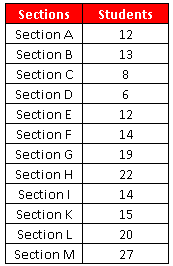# Calculate Frequency Distribution in Excel

Frequency Distribution
A Frequency Distribution is a summary of how often each value occurs by grouping values together.
For example, you have data for class sections with the number of students in each section.Excel : Frequency Distribution
There are multiple ways to calculate frequency distribution (table) with Excel.
1. With COUNTIFS Function
2. With FREQUENCY Function
Calculate Frequency Distribution in Excel

1. Enter the above data in cells B3:C15. The first row of table has headers.

The same data entered into a sheet in excel appears as follows :

2. Select the desired class intervals

3. Create a table with the columns - Class intervals, Lower limit, Upper limit and Frequency.

How to calculate lower and upper limits using excel formula -

Suppose class interval column starts from cell E5 (excluding header).

Lower limit -

Enter the following formula in cell F5 and paste it down till the last row of the table.
=MID(E5,1,FIND("-",E5,1)-1)

Upper limit -

Enter the following formula in cell G5 and paste it down till the last row of the table.
=MID(E5,FIND("-",E5,1)+1,2)

Frequency Distribution with COUNTIFS Function -

To calculate the last column of the above table, enter the following formula in cell H5 and paste it down till the last row of the table
=COUNTIFS(\$C\$4:\$C\$15,">="&F5,\$C\$4:\$C\$15,"<="&G5)Frequency Distribution with FREQUENCY Function

Create a table with the columns - Class Intervals, Upper Limit and Number of Sections

Upper Limit can be calculated with the formula below in cell F5 -
--MID(E5,FIND("-",E5,1)+1,2)
Paste the formula down till cell F9. Make sure you enter double minus (--) before MID function.

In the next step, select range G5:G9 and then enter FREQUENCY Function
=FREQUENCY(C4:C15, F5:F9)
F5:F9 refers to bins of Upper Limit.

Press CTRL SHIFT ENTER to submit the above FREQUENCY formula as it's an array formula. If it is entered correctly, you would see formula wrapped in curly braces { }

Histogram

We can prepare histogram using frequency table.

Steps:

1. Highlight the values in class intervals column (Column E) and  Frequency column (column H) of the frequency distribution table. (Holding down Ctrl key while highlighting two ranges)

2. Click on the Insert tab and select 2-D Clustered Column.

3. Remove Series1 - Select Series1 and Press Delete

1. Click on the chart.
2. Click the Layout tab under Chart Tools.
3. Click Axis Titles in the Labels group.
4. Select Primary Horizontal Axis Title and then select Title Below Axis.
5. Select Primary Vertical Axis Title and then select Title Below Axis.

Changing the axis scale

1. Select the chart.
2. Click the Layout tab under Chart Tools.
3. On the Layout tab of the ribbon, click on the Axes button.
4. Select Primary Vertical Axis >> Select More Vertical Axis Options.
5. In the Axis Options section, for Minimum, select Fixed and enter the lowest number you want on your Y-axis. In this chart, i used 0.
6. For Maximum, select Fixed, and type the number where you want the Y-axis to end. In this chart, i used 5.
7. Press Close

Related Posts
ShareDeepanshu founded ListenData with a simple objective - Make analytics easy to understand and follow. He has over 10 years of experience in data science. During his tenure, he worked with global clients in various domains like Banking, Insurance, Private Equity, Telecom and HR.

6 Responses to "Calculate Frequency Distribution in Excel "
1....or you can just use the array formula frequency

2.nicely DONE !!!

3.Thank you so much for sharing this worth able content with us. The concept taken here will be useful for my future programs and i will surely implement them in my study. Keep blogging article like this.

Ms excel training in chennai

4.Any suggestions on how to arrange in inclusive method?

5.Tq so much it was much of help

6.Thanks for sharing this knowledgeable article and very informative too. Keep sharing like this always
Traffic Lawyer Mecklenburg VA
Traffic Lawyer Harrisonburg VA

Next → ← Prev
Looks like you are using an ad blocker!

To continue reading you need to turnoff adblocker and refresh the page. We rely on advertising to help fund our site. Please whitelist us if you enjoy our content.